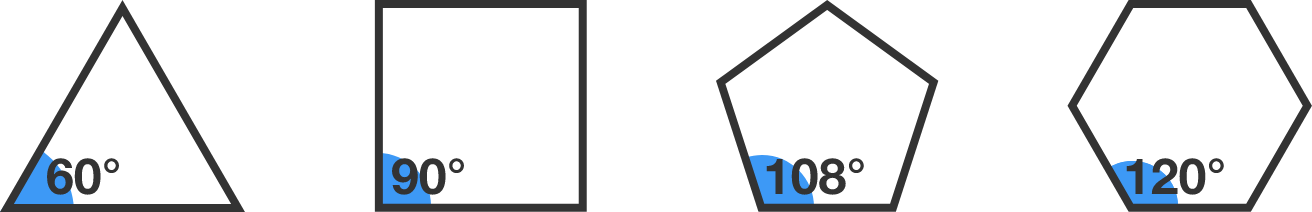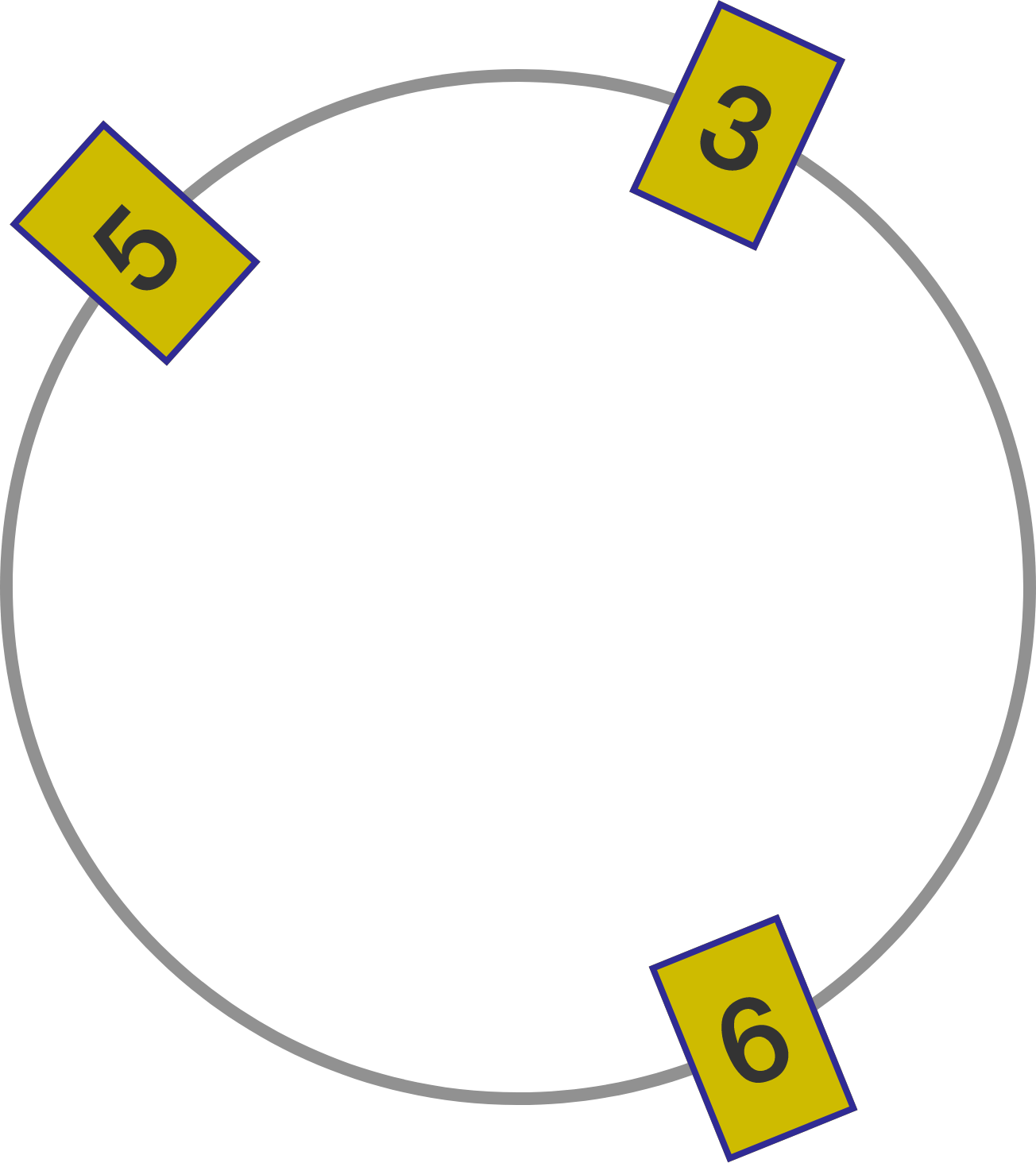# Problems of the Week

Contribute a problem

# 2018-02-05 Intermediate

A regular triangle, regular quadrilateral, regular pentagon, and regular hexagon each have interior angles measured in integer degrees.In total, how many types of regular polygons have interior angles measured in integer degrees?

How many 8-digit palindromic numbers are composite (i.e. not prime)?

Details and Assumptions:

• A palindromic number is a number that remains the same when its digits are reversed: for example, $77$ and $15851.$
• Assume that numbers are written without leading zeros. For instance, $00022000$ is not a valid 8-digit palindrome, but $10000001$ is.

$\large x=\frac{1}{2}\sqrt{7+\frac{1}{2}\sqrt{x+7}}$

$x=1$ is a solution to the equation above. Are there any other real solutions to the equation?Suppose we place $K$ distinct digits around a circle such that each pair of adjacent digits (read either clockwise or counterclockwise) forms a number that's divisible by $7.$ What's the maximum possible value of $K?$

For example, the diagram to the right illustrates a solution for $K=3.$ Note that 35, 56, and 63 are all multiples of 7.

Note: Not all pairs of digits need to be read in the same direction. Some pairs may be read clockwise and others may be read counterclockwise.

We call a positive integer $N$ square-partitionable if it can be partitioned into squares of two or more distinct positive integers, and if the sum of the reciprocals of these integers is 1. For example, $49$ is square-partitionable because $49 = 2^2 + 3^2 + 6^2\quad \text{ and }\quad \frac{1}{2} + \frac{1}{3} + \frac{1}{6} = 1.$ What is the next smallest square-partitionable integer?

Note: This problem is intended to be solved with programming.

×